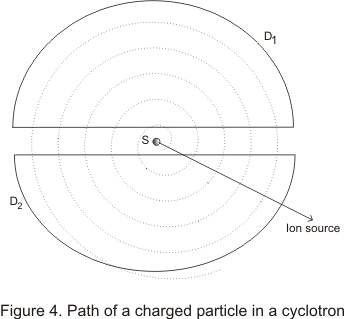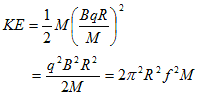# Cyclotron

## Cyclotron

• Cyclotron is a machine for producing high energy particles ,first developed by E.O.Lawrence and M.S.Livingston in 1931.Figure below shows the path of a charged particle in a cyclotronConstruction
• cyclotron consists of two horizontal D-shaped hollow metal segments D1 and D1 with a small gap between them
• These D'ees are placed in between the poles of a large electromagnet so that that magnetic field is Perpendicular to the plane of the D'ees
• The whole space inside the D'ees is evacuated to pressure of about 10-6 mm of Hg
• An ion source S is kept at the center between the D'ees
• The two D'ees are connected to the terminals of high frequency oscillating A.C circuit.This changes the charge of each D'ees several million time per sec

Theory and working

• Suppose that any instant ,alternating potential is in the direction which makes D1 positive and D2 negative
• A positive ion starting from source S will be attracted by the Dee D2
• Since Uniform magnetic field B acts at right angles to the plane of the Dees ,the positively charged ion of the charge q and mass m will move in a circular motion of radius
r=mv/qB
where v is the speed of the particle and it is constant
• After traversing half a cycle the ion comes to the edge of D2.If we adjust the frequency of the oscillator in such a way that by the time ,ion comes to the edge of D2,potential difference changes direction so as to make D1 negative and D2 positive.
• The ion will then get then attracted to D1 and its speed will increase due to acceleration
• Once inside D1 ,the ion is now in electric field free zone and again it will move in a circular path with constant speed which is higher then the previous constant speed in D2.Radius of the path in D1 will be larger then D2

• After traversing the semi -circular path in D1 ,the ion will come to the edge of D1 where if the direction of electric field changes ,it will receive additional energy
• This way the ion will continue travelling in semi circles of increasing radii every time it goes from D2 to D1 and from D1 to D2
• Time taken by the ion to traverse the semi-circular path in the Dee is given by
t=πr/v
• Thus by adjusting the magnetic field B,t can be made the same as that required to change the potential of the D1 and D2,so that positive charge ion always crosses the alternating electric field across the gap in correct phase
• Ions gain tremendous amount of energy after traversing through reversal rotation.when they come near the circumference of the Dees,an axillary electric field is used to deflect them from the circular path to eventually reach a target
• Frequency F of charged particle moving in a cyclotron is
f=ω/2π =υ/2πr=Bυ/2πm                   -- (10)

where υ=1/2t
• If f and B are adjusted to keep charged ion always in phase each time ,the ion crosses the gap .it receive additional energy and at the same time it describes a flat spiral of increasing radius
• KE of ion emerging from the cyclotron if R is radius of the D'ees is• Above relation shows that the maximum energy attained by the ion is limited by the radius R of the D'ees ,magnetic field B or the frequency f
• Maximum energy acquired by the charged particle in a particular cyclotron is independent of the alternating potential i.e when the voltage is small the ion makes a large number of the turns before reaching the periphery and for the large voltage number of turns is small.Total energy remains the same in both the cases,provided both B and R are unchanged
• These days cyclotron are not in wide use but others based on principle of cyclotron are used